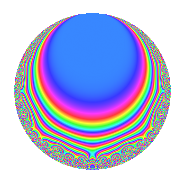Properties

 Label 15.4.eLevel 15 Weight 4 Character orbit e Rep. character $$\chi_{15}(2,\cdot)$$ Character field $$\Q(\zeta_{4})$$ Dimension 8 Newforms 1 Sturm bound 8 Trace bound 0

Related objects

Defining parameters

 Level: $$N$$ = $$15 = 3 \cdot 5$$ Weight: $$k$$ = $$4$$ Character orbit: $$[\chi]$$ = 15.e (of order $$4$$ and degree $$2$$) Character conductor: $$\operatorname{cond}(\chi)$$ = $$15$$ Character field: $$\Q(i)$$ Newforms: $$1$$ Sturm bound: $$8$$ Trace bound: $$0$$

Dimensions

The following table gives the dimensions of various subspaces of $$M_{4}(15, [\chi])$$.

Total New Old
Modular forms 16 16 0
Cusp forms 8 8 0
Eisenstein series 8 8 0

Trace form

 $$8q$$ $$\mathstrut -\mathstrut 6q^{3}$$ $$\mathstrut -\mathstrut 12q^{6}$$ $$\mathstrut -\mathstrut 16q^{7}$$ $$\mathstrut +\mathstrut O(q^{10})$$ $$8q$$ $$\mathstrut -\mathstrut 6q^{3}$$ $$\mathstrut -\mathstrut 12q^{6}$$ $$\mathstrut -\mathstrut 16q^{7}$$ $$\mathstrut -\mathstrut 100q^{10}$$ $$\mathstrut +\mathstrut 132q^{12}$$ $$\mathstrut +\mathstrut 68q^{13}$$ $$\mathstrut +\mathstrut 90q^{15}$$ $$\mathstrut +\mathstrut 284q^{16}$$ $$\mathstrut -\mathstrut 240q^{18}$$ $$\mathstrut -\mathstrut 492q^{21}$$ $$\mathstrut -\mathstrut 500q^{22}$$ $$\mathstrut -\mathstrut 220q^{25}$$ $$\mathstrut +\mathstrut 702q^{27}$$ $$\mathstrut +\mathstrut 508q^{28}$$ $$\mathstrut +\mathstrut 660q^{30}$$ $$\mathstrut +\mathstrut 616q^{31}$$ $$\mathstrut -\mathstrut 240q^{33}$$ $$\mathstrut -\mathstrut 804q^{36}$$ $$\mathstrut -\mathstrut 1156q^{37}$$ $$\mathstrut -\mathstrut 600q^{40}$$ $$\mathstrut +\mathstrut 540q^{42}$$ $$\mathstrut +\mathstrut 548q^{43}$$ $$\mathstrut +\mathstrut 180q^{45}$$ $$\mathstrut +\mathstrut 736q^{46}$$ $$\mathstrut -\mathstrut 1116q^{48}$$ $$\mathstrut -\mathstrut 852q^{51}$$ $$\mathstrut +\mathstrut 224q^{52}$$ $$\mathstrut +\mathstrut 460q^{55}$$ $$\mathstrut +\mathstrut 684q^{57}$$ $$\mathstrut +\mathstrut 60q^{58}$$ $$\mathstrut +\mathstrut 540q^{60}$$ $$\mathstrut +\mathstrut 16q^{61}$$ $$\mathstrut +\mathstrut 1428q^{63}$$ $$\mathstrut +\mathstrut 2040q^{66}$$ $$\mathstrut +\mathstrut 404q^{67}$$ $$\mathstrut -\mathstrut 2220q^{70}$$ $$\mathstrut -\mathstrut 1800q^{72}$$ $$\mathstrut -\mathstrut 2512q^{73}$$ $$\mathstrut -\mathstrut 2910q^{75}$$ $$\mathstrut -\mathstrut 1488q^{76}$$ $$\mathstrut -\mathstrut 360q^{78}$$ $$\mathstrut +\mathstrut 288q^{81}$$ $$\mathstrut +\mathstrut 2800q^{82}$$ $$\mathstrut +\mathstrut 4940q^{85}$$ $$\mathstrut -\mathstrut 1680q^{87}$$ $$\mathstrut +\mathstrut 2460q^{88}$$ $$\mathstrut +\mathstrut 600q^{90}$$ $$\mathstrut -\mathstrut 1304q^{91}$$ $$\mathstrut +\mathstrut 3408q^{93}$$ $$\mathstrut +\mathstrut 4164q^{96}$$ $$\mathstrut +\mathstrut 1904q^{97}$$ $$\mathstrut +\mathstrut O(q^{100})$$

Decomposition of $$S_{4}^{\mathrm{new}}(15, [\chi])$$ into irreducible Hecke orbits

Label Dim. $$A$$ Field CM Traces $q$-expansion
$$a_2$$ $$a_3$$ $$a_5$$ $$a_7$$
15.4.e.a $$8$$ $$0.885$$ 8.0.$$\cdots$$.8 None $$0$$ $$-6$$ $$0$$ $$-16$$ $$q-\beta _{3}q^{2}+(-1-\beta _{2}+\beta _{5})q^{3}+(\beta _{2}+\cdots)q^{4}+\cdots$$